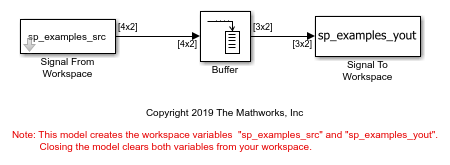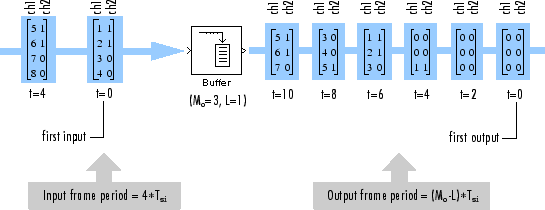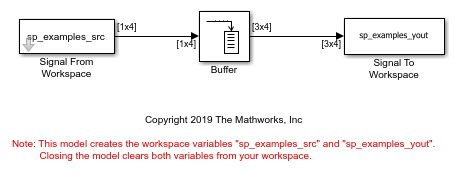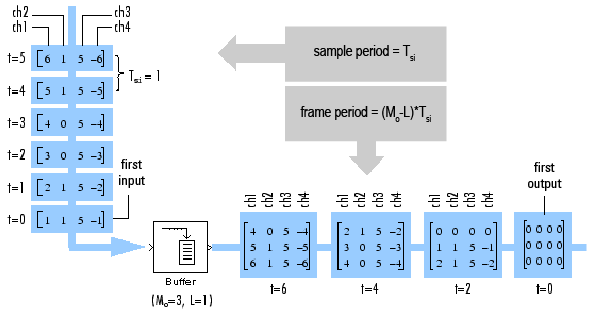# Buffering Input with Overlap

### Buffering Two-Channel Input with Overlap

In the `ex_buffer_tut4` model, the Buffer block uses a one-sample overlap and rebuffers a signal with the frame size of 4 into a signal with the frame size of 3.

Open and run the model.

```two_channel_model = "ex_buffer_tut4"; open_system(two_channel_model); sim(two_channel_model); ```The following diagram illustrates the inputs and outputs of the Buffer block.The output is delayed by eight samples. This latency occurs because of the parameter settings chosen in this model, and because the model is running in Simulink in the multitasking mode. The first eight output samples therefore adopt the value specified in the Initial conditions parameter, which in this case is zero. You can use the rebuffer_delay function to determine the latency of the Buffer block for any combination of frame size and overlap values.

### Buffering Four-Channel Input with Overlap

The ex_buffer_tut3 model buffers a 1-by-4 input signal using an output buffer size of 3 and a buffer overlap of 1. The buffered output is a 3-by-4 signal.

Open and run the model.

```four_channel_model = "ex_buffer_tut3"; open_system(four_channel_model); sim(four_channel_model); ```This diagram illustrates the inputs and outputs of the Buffer block.The input vectors do not begin appearing at the output until the second row of the second matrix. This is due to latency in the Buffer block. The first output matrix (all zeros in this example) reflects the value of the Initial conditions parameter, while the first row of zeros in the second output is a result of the one-sample overlap between consecutive output frames.

You can use the rebuffer_delay function with a frame size of 1 to precisely compute the delay (in samples).

```d = rebuffer_delay(1,3,1) ```
```d = 4 ```

This number agrees with the four samples of delay (zeros) per channel shown in the previous figure.

## SupportGet trial now